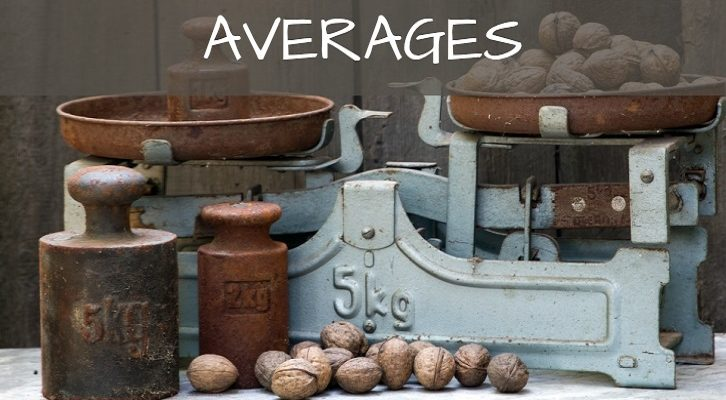# Mean median – Two more questions

Have given below two more questions on Averages.

1. Consider three classes A, B and C with 20, 30 and 50 students respectively. The averages score in maths of students in class B is 16 more than that of those in class C and the average score of those in class A is 2 more than the overall average of scores in A, B and C. What is the difference between average of class C and class A

2. Natural numbers 1 to 25 (both inclusive) are split into 5 groups of 5 numbers each. The medians of these 5 groups are A, B, C, D and E. If the average of these medians is m, what are the smallest and the largest values m can take?